Back# Data Visualization Python

09 March 2021

This is a beginner's tutorial for enthusiasts new to pandas or data visualization. The tutorial assumes little or no knowledge of python. Before we start, here is an overview of the libraries and setups we will use in the tutorial.

Pandas is an open-source Python library that lets you manipulate data quickly, including storing it in various forms. Jupyter Notebook is an open-source web application that allows you to create documents and programs in multiple programming languages.

Today we will look at how to get, change and store forex data from a JSON API and, during the process, learn about Pandas, DataFrame, and Jupyter Notebook.

Open your favorite browser and navigate to https://jupyter.org/try Select the "Classic Notebook" link as shown below.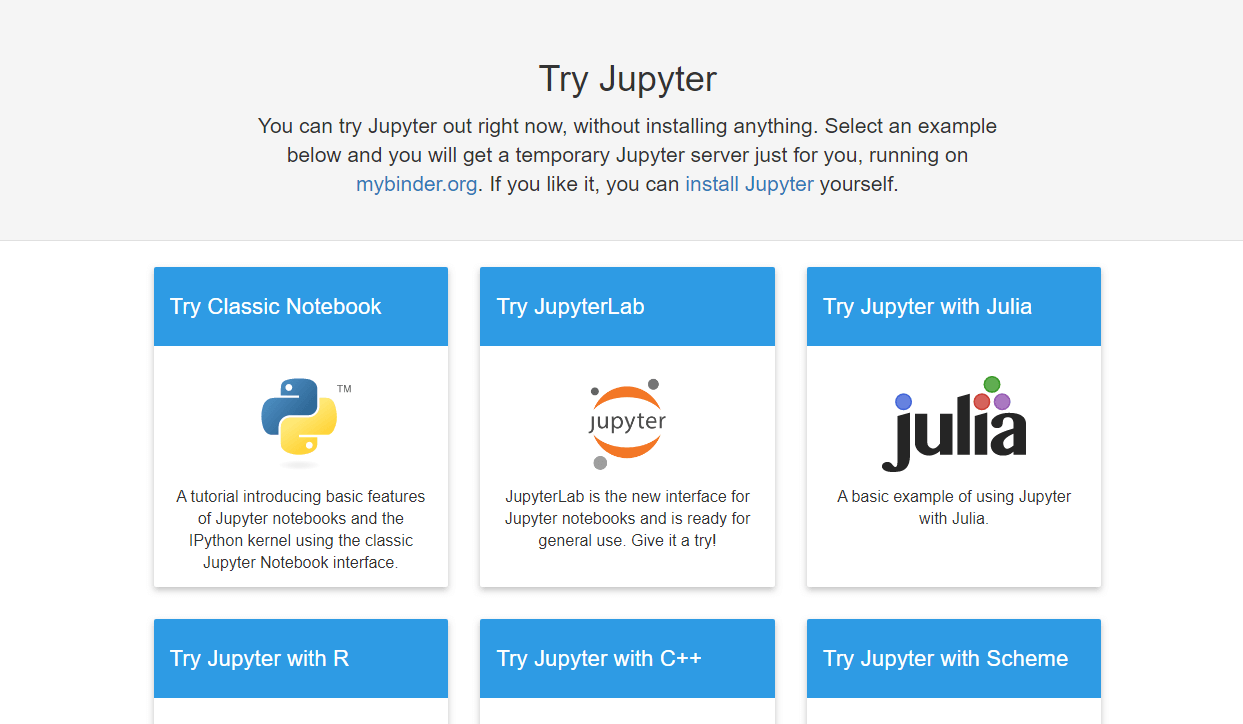Wait a few seconds, and the link will launch a Jupyter Notebook for you in the browser.

Once you have started the notebook, let us get an API key from https://marketdata,tradermade.com/signup. The account is free for up to 1000 requests a month. Once you have registered an account, you will find your API Key under the myAccount page.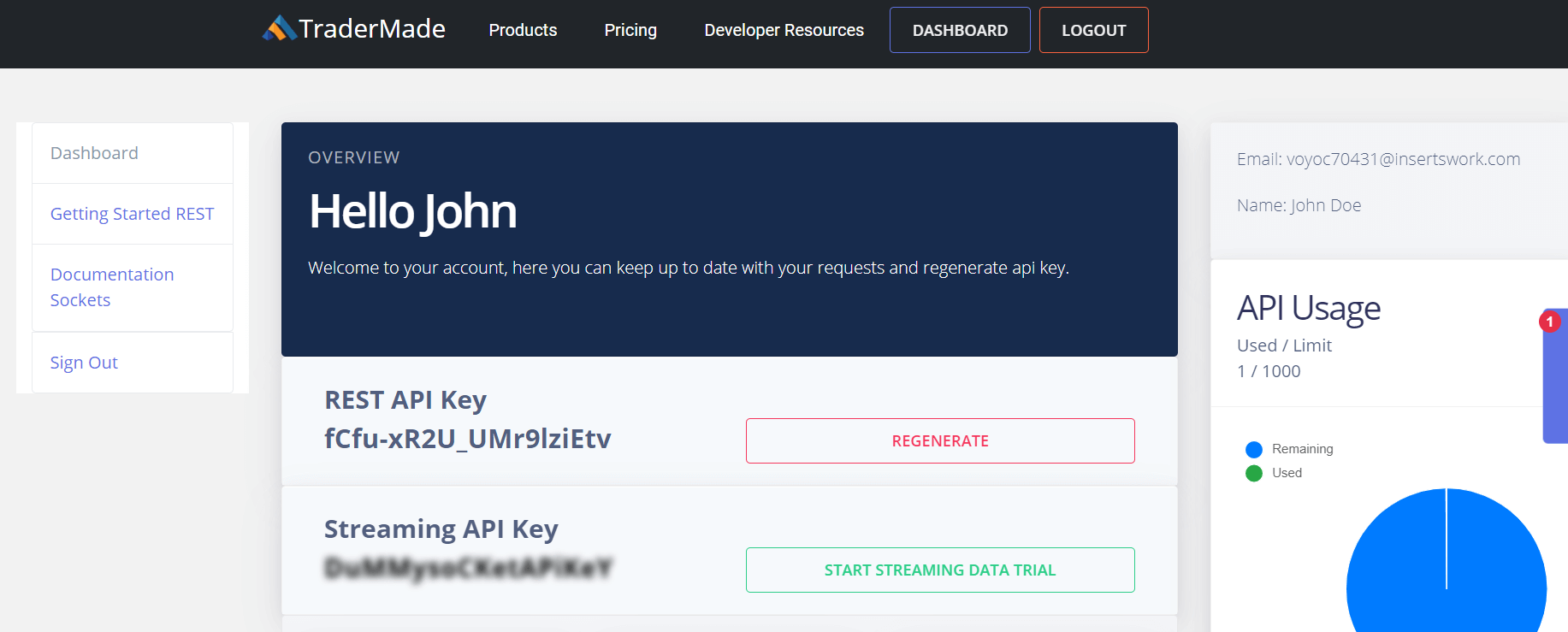Now that we have the API Key, we can get started.

Click on the scissor sign (third from the left under Edit) three times. This clears out the welcome content so we can start coding.

#### Now let us write some code.

In the cell, write the following command and click run:

```
import pandas as pd

```

This command imports the Pandas Data Frame for use in our notebook. You can read more about Pandas here https://pandas.pydata.org.

In the next cell, type or copy the following variables. Here we are setting up into settings we will pass in the data request. Do not forget to substitute your api_key.

```
currency = "EURUSD"
api_key = “paste your api key here”
start_date = "2019-10-01"
end_date= "2019-10-30"
format = "records"
fields = "ohlc"

```

Copy the following code. The first line of code makes a request to the data server and formats the returned JSON data as a Pandas DataFrame, and the second line of code sets the index for the data to the date field. So, it is displayed in date order, and the third line of code - "df" - outputs the data frame in a presentable format.

```
df = df.set_index("date")
df

```

Once you can copy the code and substitute your api_key, you can run the program. You will get the following. The program has requested data from the data server, written it into Pandas DataFrame format, and output the data in a table format.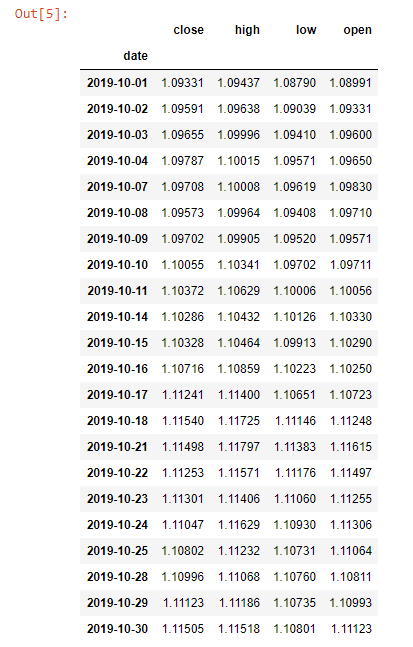As you can see, we have received data for the specified period in a data frame similar to a table. But the cool thing about Pandas is their ability to manipulate information and store data in various forms.

Before we dig deeper, let us look at some basic commands:

df.head() - This will give us the first 5 lines of data

df.tail() - This will give us the last 5 lines of data

#### Let us plot our data as a line chart.

We can use the command df.plot(kind='line'), and you will get the following chart. Yes, it really is that simple.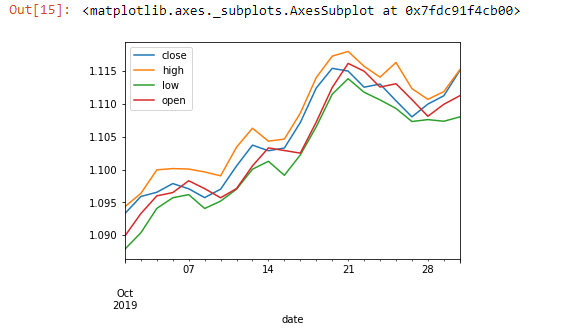The above command helps us visualize the data set. Now let us do something with our data set. It will require some effort in Excel. Let us calculate the 5-day moving average:

```
df['5_day_MA'] =  df.close.rolling(5).mean()
df['5_day_volatility'] = df.close.rolling(5).std()
df = df.dropna()

```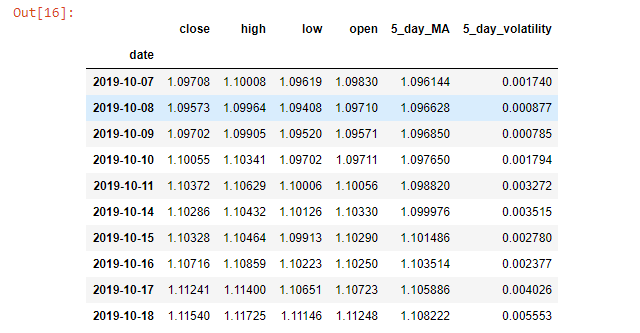As you can see, the dropna() function drops the value that is N/A. Because we need at least 5 close days to get the first moving average. Our data set is now from 07/10/2019.

The plotting function can now let us see if the current data we pulled shows if the volatility is going up or down.

```
df["5_day_volatility"].plot(title="EURUSD Volatility")

```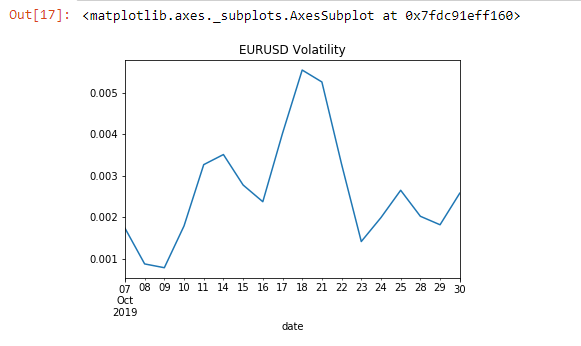So far, we have looked at how a single data set works. Now let's use the API to compare two currencies:

```
currency = "EURUSD,GBPUSD"
fields = "close"
"&end_date="+end_date+"&format="+format+"&fields="+fields)
df = df.set_index("date")

```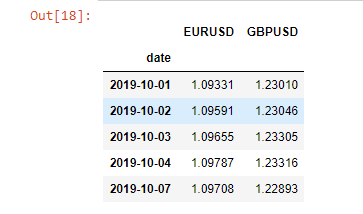We will first calculate the percentage change for the currencies before seeing if there is any correlation between the two and then plot it.

```
df = df.pct_change(periods=1)
df = df.dropna()
df.corr()

```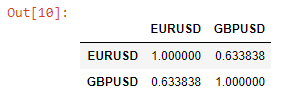The above function lets us see the daily correlation between EURUSD and GBPUSD over the whole data set. Next up, we will see how this relationship is changing over time.

```
df["EURUSD_GBPUSD"] = df["EURUSD"].rolling(window=5).corr(other= df["GBPUSD"])
df = df.dropna()
df["EURUSD_GBPUSD"].plot(title="5 day correlation between EURUSD and GBPUSD")

```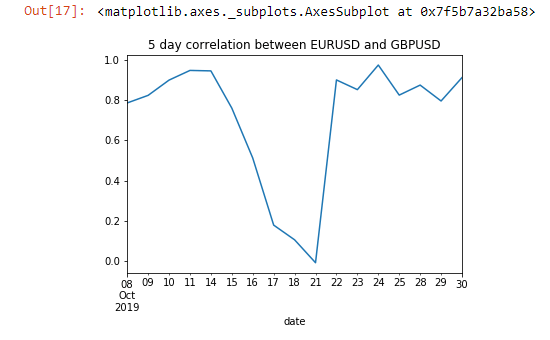We can see a change in this correlation between the 15th to the 21st of October. If we have to save data in a CSV or JSON format, we can also do that by:

```
df.to_json(‘mydata.json’)
df.to_csv(‘mydata.csv’)

```

Now if you click: File > open > myfile.json, you can see that the data is available. Also, if you want to download, click the file and click download.

If you have any queries or questions, we are more than happy to answer queries via live chat or support@tradermade.com